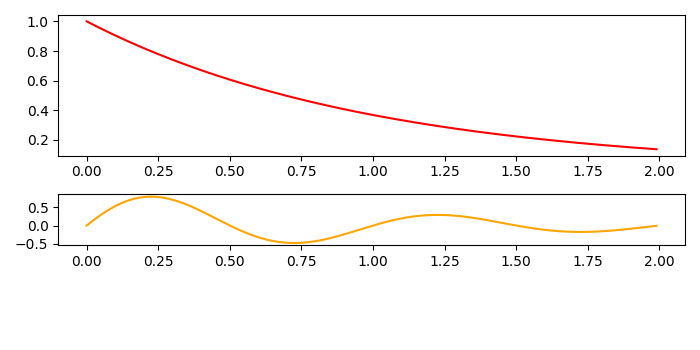How to sharex when using subplot2grid?

MatplotlibPythonData Visualization

To sharex when using subplot2grid, we can take the following steps −

• Create random data, t, x, y1 and y2 using numpy.

• Create a new figure or activate an existing figure using figure() method.

• Create a subplot at a specific location inside a regular grid with colspan=3 and rowspan=2.

• Create a subplot at a specific location inside a regular grid with colspan=3 and sharex=ax1 (step 3).

• Plot curve using t and y1 and y2 using plot() method.

• To display the figure, use show() method.

Example

import numpy as np
from matplotlib import pyplot as plt
plt.rcParams["figure.figsize"] = [7.00, 3.50]
plt.rcParams["figure.autolayout"] = True
t = np.arange(0.0, 2.0, 0.01)

x = np.sin(2 * np.pi * t)
y1 = np.exp(-t)
y2 = x * y1
fig = plt.figure()
ax1 = plt.subplot2grid((4, 3), (0, 0), colspan=3, rowspan=2)
ax2 = plt.subplot2grid((4, 3), (2, 0), colspan=3, sharex=ax1)

ax1.plot(t, y1, c='red')
ax2.plot(t, y2, c='orange')

plt.tight_layout()

plt.show()

Output